# mne.combine_evoked#

mne.combine_evoked(all_evoked, weights)[source]#

Merge evoked data by weighted addition or subtraction.

Each `Evoked` in `all_evoked` should have the same channels and the same time instants. Subtraction can be performed by passing `weights=[1, -1]`.

Warning

Other than cases like simple subtraction mentioned above (where all weights are -1 or 1), if you provide numeric weights instead of using `'equal'` or `'nave'`, the resulting `Evoked` object’s `.nave` attribute (which is used to scale noise covariance when applying the inverse operator) may not be suitable for inverse imaging.

Parameters:
all_evoked

The evoked datasets.

weights`list` of `float` | ‘equal’ | ‘nave’

The weights to apply to the data of each evoked instance, or a string describing the weighting strategy to apply: `'nave'` computes sum-to-one weights proportional to each object’s `nave` attribute; `'equal'` weights each `Evoked` by `1 / len(all_evoked)`.

Returns:
evoked`Evoked`

The new evoked data.

Notes

New in version 0.9.0.

## Examples using `mne.combine_evoked`#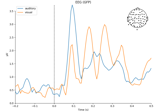Overview of MEG/EEG analysis with MNE-Python

Overview of MEG/EEG analysis with MNE-Python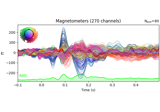Working with CTF data: the Brainstorm auditory dataset

Working with CTF data: the Brainstorm auditory dataset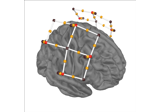Preprocessing functional near-infrared spectroscopy (fNIRS) data

Preprocessing functional near-infrared spectroscopy (fNIRS) data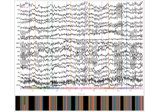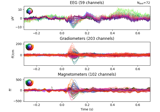The Evoked data structure: evoked/averaged data

The Evoked data structure: evoked/averaged data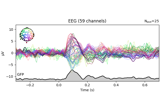EEG analysis - Event-Related Potentials (ERPs)

EEG analysis - Event-Related Potentials (ERPs)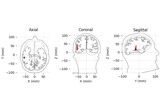Source localization with equivalent current dipole (ECD) fit

Source localization with equivalent current dipole (ECD) fit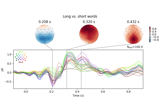Visualising statistical significance thresholds on EEG data

Visualising statistical significance thresholds on EEG data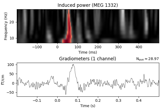Non-parametric between conditions cluster statistic on single trial power

Non-parametric between conditions cluster statistic on single trial power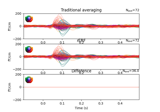Regression on continuous data (rER[P/F])

Regression on continuous data (rER[P/F])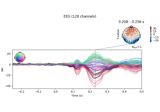Single trial linear regression analysis with the LIMO dataset

Single trial linear regression analysis with the LIMO datasetFrom raw data to dSPM on SPM Faces dataset

From raw data to dSPM on SPM Faces dataset